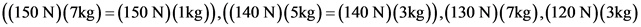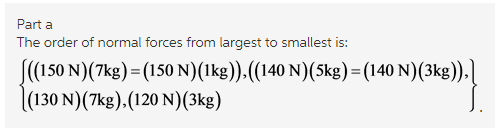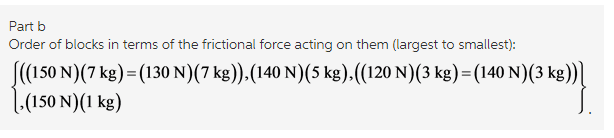# Rank the boxes on the basis of the magnitude of the normal force acting on them

Rank the boxes on the basis of the magnitude of the normal force acting on them.

Concepts and reason
The concept used here is frictional forces and equilibrium of forces.
The normal force is the component of the force which is perpendicular to the surface of the contact. The force exerted on an object on the wall or floor, preventing the object from falling.
Consider a person standing still on the ground, in that case ground reaction force reduces to the normal force.
Here, first observe the forces opposite to the surface against which the blocks have been pushed to find the normal and frictional forces.
After that compare all the values of frictional and normal forces.

Fundamentals
Friction is the force resisting the relative motion of solid surfaces, layers, fluid and material elements sliding against each other.
It is a non-conservative force. The work done against friction is path dependent. For frictional surface loss is in the form of heat. Thus mechanical energy is not conserved.

(a)
The force perpendicular to the surface against which the block is been pressed, is the normal force.
The expression of the normal force if there is no nay inclination in the system then expression is as follows:
N = mg
Here,Nis the normal force,mis the mass of the object and g is the acceleration due to the gravity.
From the provided question external force acts on the body. Therefore, the rank of the magnitude of the normal force is equal to,Explanation:
The block having the largest force perpendicular to its surface will have the largest normal force regardless of its mass. If the external force acts in the body then the normal force is equal to the magnitude of the heights external applied force.

(b)
The frictional forces acting on the blocks is equal to the weight of the blocks. Larger the mass of the object, larger is the frictional force.
Order of blocks in terms of the frictional force acting on them (largest to smallest):Explanation:
The frictional force which acts parallel to the surface against which the block is pushed is equal to the weight of the block. Hence, the block with highest weight has the highest frictional force acting on it.